# Site map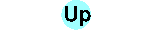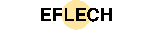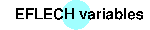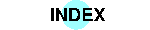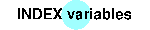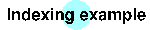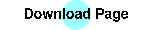## EFLECH Control File

The diagram deconvolution with EFLECH is controlled by a simple text file with the file extension 'sav'. A list and extended explanation of the variables used is available.

By means of the control file for the potassiumdichromate sample (`K2Cr2O7.sav`) the necessary elements will be explained here.

First, the instrumental function (calculated with GEOMET and MAKEGEQ, see BGMN site) has to be provided:

```VERZERR=rd7ff31.geq
```
Then the measuring value file is needed:
```VAL=k2cr2o7.val
```

This file is in the APX-63 format, a simple text format from the SEIFERT-FPM diffractometers. EFLECH can process files with the extension 'rd' (Philips APD) and 'raw' (SIEMENS Diffrac-AT) as well. If you want to use other formats, you have to convert it into one of the above.

If a secondary graphite monochromator was used, an appropriate polarisation factor is (26.6ˆ is the 2Θ angle of the graphite crystal; without monochromator use 'POL=1', which is the default):

```pi=2*acos(0)
POL=sqr(cos(26.6*pi/180))
```
I do not recommend to calculate a whole diagram with EFLECH (this is possible, of course!). Calculation of a whole diagram would take a large amount of CPU time for the hundreds of peak search steps. Therefore, I have developed the auxiliary program TEIL. TEIL automatically divides the whole diagram into some parts separated by background intervals of sufficient length. TEIL uses the variables
```OUTPUTMASK=k2cr2o7-\$
```
for generating items in the `*.sav`-file. The character `\$` is replaced by the actual numbers of the diagram's parts, which should be handled separately with EFLECH. TEIL automatically determines the rank of the background polynomial (of course, you can set it manual with `RU=...`). TEIL reports the count of parts on the screen.

For sucessful indexing, you may try the following entry in the `k2cr2o7.sav` file:

```TEST=4DNU
```
That restricts EFLECH during peak seach, it will only use peaks with two width parameters (`RP=4`), delete peaks, insert new peaks and simplify background polynomial. This strategy will minimize the "satellite peaks" count as found by EFLECH, INDEX will found better solutions and will be faster.

The calculation is started by the commands

```teil k2cr2o7
eflech k2cr2o7
```
, and for each added/modified/delted peak a line is printed on the screen.

If the program doesn't start: This is the first FAQ!

## Peak listing

A peak listing of the whole diagram may be printed using the command
```OUTPUT k2cr2o7
```
which has some additional features not described in the BGMN pages:
• OUTPUT scans automaticaly all partial diagrams and prints a whole peak list for the whole diagram.
• In addition to the BGMN delivered `*.par`-files, EFLECH delivers the whole covariance matrice of the peak parameters at the end of the `*.par`-files. OUTPUT prints error informations (1σ) according to this covariance matrice.

## Diagram output

Diagram output is possible using the command
```SHOW k2cr2o7 x x x x part-No
```
You may visit partial diagrams, only. `part-No` must be the number of the wanted diagram part. This is an extension of the SHOW command, which is already described on the BGMN sites. Therefore, `x x x x` may be replaced by angular/intensity ranges as described there.